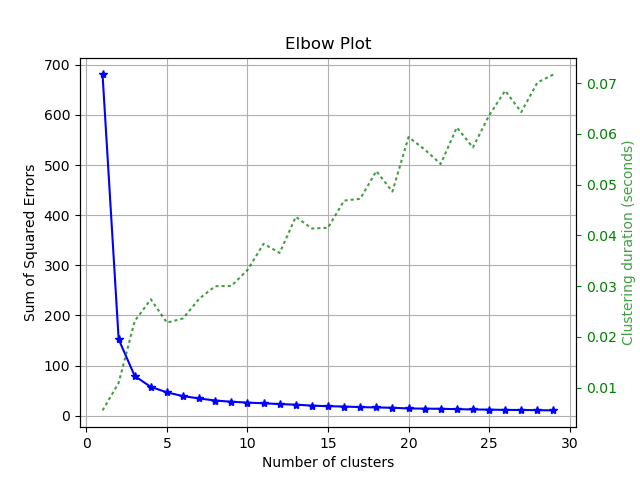# Clusterer Module (API Reference)¶

The `scikitplot.cluster` module includes plots built specifically for scikit-learn clusterer instances e.g. KMeans. You can use your own clusterers, but these plots assume specific properties shared by scikit-learn estimators. The specific requirements are documented per function.

`scikitplot.cluster.``plot_elbow_curve`(clf, X, title='Elbow Plot', cluster_ranges=None, n_jobs=1, show_cluster_time=True, ax=None, figsize=None, title_fontsize='large', text_fontsize='medium')

Plots elbow curve of different values of K for KMeans clustering.

Parameters: clf – Clusterer instance that implements `fit`,``fit_predict``, and `score` methods, and an `n_clusters` hyperparameter. e.g. `sklearn.cluster.KMeans` instance X (array-like, shape (n_samples, n_features)) – Data to cluster, where n_samples is the number of samples and n_features is the number of features. title (string, optional) – Title of the generated plot. Defaults to “Elbow Plot” cluster_ranges (None or `list` of int, optional) – List of n_clusters for which to plot the explained variances. Defaults to `range(1, 12, 2)`. n_jobs (int, optional) – Number of jobs to run in parallel. Defaults to 1. show_cluster_time (bool, optional) – Include plot of time it took to cluster for a particular K. ax (`matplotlib.axes.Axes`, optional) – The axes upon which to plot the curve. If None, the plot is drawn on a new set of axes. figsize (2-tuple, optional) – Tuple denoting figure size of the plot e.g. (6, 6). Defaults to `None`. title_fontsize (string or int, optional) – Matplotlib-style fontsizes. Use e.g. “small”, “medium”, “large” or integer-values. Defaults to “large”. text_fontsize (string or int, optional) – Matplotlib-style fontsizes. Use e.g. “small”, “medium”, “large” or integer-values. Defaults to “medium”. The axes on which the plot was drawn. ax (`matplotlib.axes.Axes`)

Example

```>>> import scikitplot as skplt
>>> kmeans = KMeans(random_state=1)
>>> skplt.cluster.plot_elbow_curve(kmeans, cluster_ranges=range(1, 30))
<matplotlib.axes._subplots.AxesSubplot object at 0x7fe967d64490>
>>> plt.show()
```